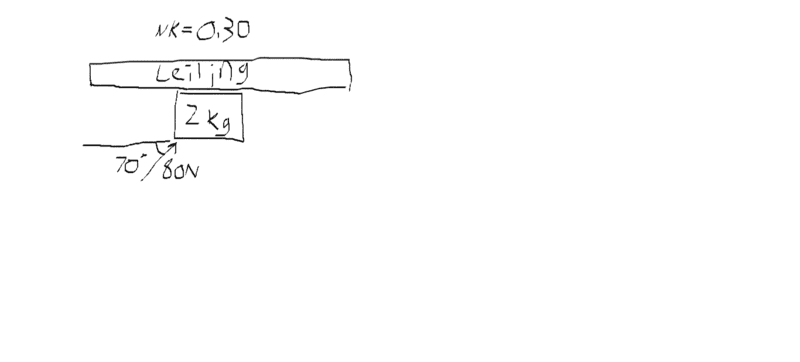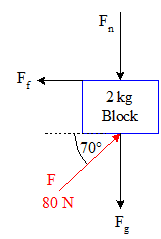# Free body diagram on a block pushed against a ceiling

## Homework Statement

A 2kg block is being pushed along a ceiling with friction by an angled force of 70 degrees. I'm asked only for the free body diagram.

## The Attempt at a Solution

I figured both the gravitational and normal force would point downwards, but the force applied at an angle(figure an angle someone would apply by pushing with arms) I'm confused on how to draw on the diagram.

Mentor

## Homework Statement

A 2kg block is being pushed along a ceiling with friction by an angled force of 70 degrees. I'm asked only for the free body diagram.
70 degrees with respect to what?
I figured both the gravitational and normal force would point downwards, but the force applied at an angle(figure an angle someone would apply by pushing with arms) I'm confused on how to draw on the diagram.
What is the direction of the force? That's the direction the force vector will make with the block.#### Attachments

I forgot to add that friction plays a role. I'm just confused on how to set up the free body diagram

Mentor
Your applied force looks good. Now you need to add gravitational force and frictional force vectors. In which directions do they act?

Mentor
Okay, it looks like you've got all the forces represented. That's good. What I would suggest, however, is that they be presented a bit more aesthetically. Often, leaving in the depiction of body being acted on makes things easier to recognize and process. So maybe something like this:The placements of the force vectors around the block are not as important as getting their directions right, but making your diagram easy to read (especially by markers!) is at least a good portion of the battle#### Attachments

•